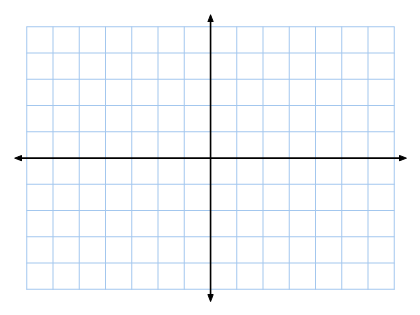# Coordinate Plane Check

10 Questions | Total Attempts: 69SettingsCoordinate plane is a plane spanned by the x-axis and y-axis in which the coordinates of a point are its distances from two intersecting perpendicular axes. Take this assessment test to assess your knowledge of coordinate plane.

Related Topics
• 1.
The horizontal number line on a coordinate plane is known as the...
• A.

Y-axis

• B.

X-axis

• C.

Z-axis

• D.

W-axis

• 2.
The vertical number line on a coordinate plane is known as the...
• A.

Y-axis

• B.

X-axis

• C.

Z-axis

• D.

W-axis

• 3.
The point at which the two axes intersects is called...
• A.

Origin

• B.

Base

• C.

Curve

• D.

Plane

• 4.
How many quadrants are in a coordinate plane?
• A.

4

• B.

3

• C.

2

• D.

5

• 5.
In what quadrant are both x and y positive?
• A.

1

• B.

2

• C.

3

• D.

4

• 6.
In what quadrant are both x and y negative?
• A.

1

• B.

2

• C.

3

• D.

4

• 7.
Where would the value of x be 0?
• A.

On the x axis

• B.

On the y axis

• C.

• D.

• 8.
A flat surface that extends forever in two dimensions, but has no thickness is...
• A.

Board

• B.

Plane

• C.

Angle

• D.

Line

• 9.
In writing coordinates, which one comes first?
• A.

D

• B.

X

• C.

Y

• D.

B

• 10.
A plane with x and y-axis defined is referred to as...
• A.

Triangle

• B.

Cartesian plane

• C.

Curve

• D.

Plot